Home

# Non-rigid quartic$3$ -folds

## Abstract

Let$X\subset \mathbb{P}^{4}$ be a terminal factorial quartic$3$ -fold. If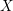$X$ is non-singular,$X$ is birationally rigid, i.e. the classical minimal model program on any terminal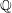$\mathbb{Q}$ -factorial projective variety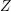$Z$ birational to$X$ always terminates with$X$ . This no longer holds when$X$ is singular, but very few examples of non-rigid factorial quartics are known. In this article, we first bound the local analytic type of singularities that may occur on a terminal factorial quartic hypersurface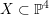$X\subset \mathbb{P}^{4}$ . A singular point on such a hypersurface is of type$cA_{n}$ ($n\geqslant 1$ ), or of type$cD_{m}$ ($m\geqslant 4$ ) or of type$cE_{6},cE_{7}$ or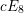$cE_{8}$ . We first show that if$(P\in X)$ is of type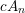$cA_{n}$ ,$n$ is at most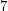$7$ and, if$(P\in X)$ is of type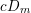$cD_{m}$ ,$m$ is at most$8$ . We then construct examples of non-rigid factorial quartic hypersurfaces whose singular loci consist (a) of a single point of type$cA_{n}$ for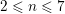$2\leqslant n\leqslant 7$ , (b) of a single point of type$cD_{m}$ for$m=4$ or$5$ and (c) of a single point of type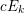$cE_{k}$ for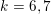$k=6,7$ or$8$ .

• # Send article to Kindle

Note you can select to send to either the @free.kindle.com or @kindle.com variations. ‘@free.kindle.com’ emails are free but can only be sent to your device when it is connected to wi-fi. ‘@kindle.com’ emails can be delivered even when you are not connected to wi-fi, but note that service fees apply.

Find out more about the Kindle Personal Document Service.

Non-rigid quartic$3$-folds Available formats × # Send article to Dropbox To send this article to your Dropbox account, please select one or more formats and confirm that you agree to abide by our usage policies. If this is the first time you use this feature, you will be asked to authorise Cambridge Core to connect with your <service> account. Find out more about sending content to Dropbox. Non-rigid quartic$3$ -folds
Available formats
×

# Send article to Google Drive

To send this article to your Google Drive account, please select one or more formats and confirm that you agree to abide by our usage policies. If this is the first time you use this feature, you will be asked to authorise Cambridge Core to connect with your <service> account. Find out more about sending content to Google Drive.

## Metrics

### Full text viewsFull text views reflects the number of PDF downloads, PDFs sent to Google Drive, Dropbox and Kindle and HTML full text views.

Total number of HTML views: 0
Total number of PDF views: 0 *Loading metrics...

### Abstract viewsAbstract views reflect the number of visits to the article landing page.

Total abstract views: 0 *Loading metrics...

* Views captured on Cambridge Core between <date>. This data will be updated every 24 hours.

Usage data cannot currently be displayed